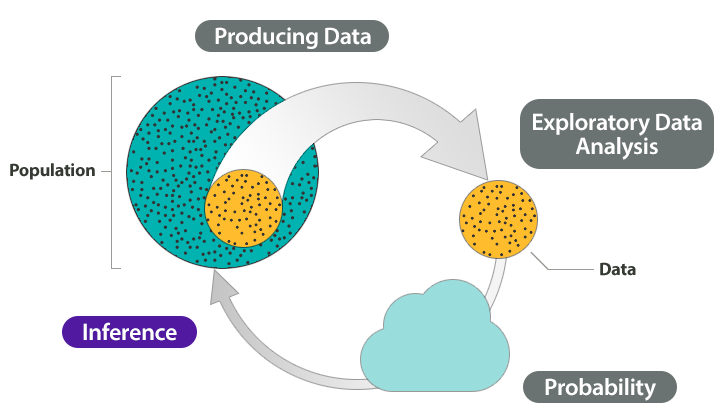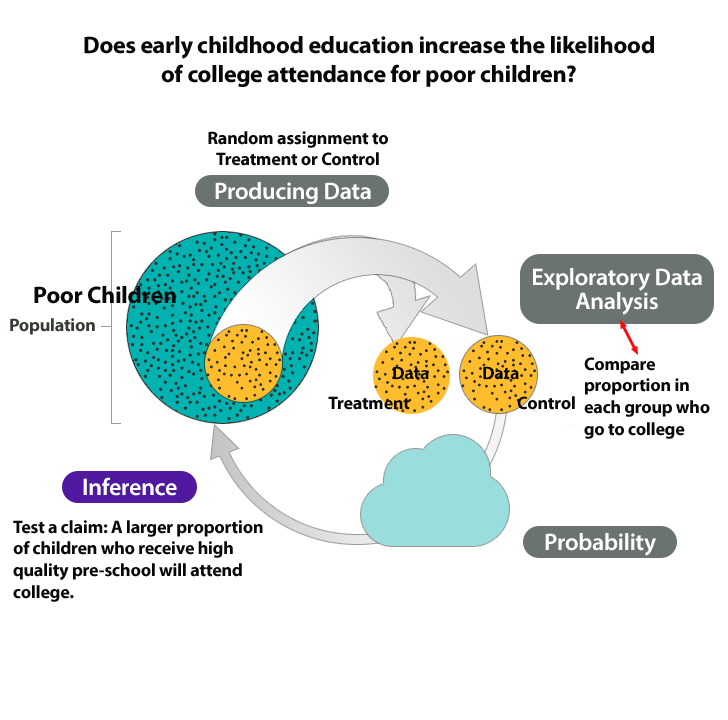## Why It Matters: Inference for Two Proportions

### Learning Objectives

• Recognize when to use a hypothesis test or a confidence interval to compare two population proportions or to investigate a treatment effect for a categorical variable.
• Determine if a study involving two proportions is an experiment or an observational study.

In previous modules, we learned to make inferences about a population proportion. In particular, we learned the following:

• Random samples vary. When we use a sample proportion to make an inference about a population proportion, there is uncertainty. For this reason, inference involves probability.
• Under certain conditions, we can model the variability in sample proportions with a normal curve. We use the normal curve to make probability-based decisions about population values.
• We can estimate a population proportion with a confidence interval. The confidence interval is an actual sample proportion with a margin of error. We state our confidence in the accuracy of these intervals using probability.
• We can test a hypothesis about a population proportion using an actual sample proportion. Again, we base our conclusion on probability using a P-value. The P-value describes the strength of our evidence in rejecting a hypothesis about the population.

In Inference for Two Proportions, we continue to work with categorical data, so we continue to use proportions. But now we make inferences that compare two populations (or two treatments).

As an overview, consider again the Big Picture of Statistics.Here we discuss the four steps in a statistical investigation for situations from Module 9.

1. Produce Data: Determine what to measure, then collect the data. In this module, we collect categorical data from two samples. In an observational study, we begin with two populations and randomly select a sample from each population. In an experiment, we randomly assign individuals to two treatments. The use of random selection or random assignment allows us to view the samples as independent. This means we assume that the variable values from one sample do not influence the values for the other sample.
2. Exploratory Data Analysis: Analyze and summarize the data. We are working with categorical data, so from each sample, we compute a sample proportion. To compare the two samples, we subtract the proportions. When we conduct inference in the next step, our goal is to to determine if the actual difference in the sample proportions is significantly different from what we expect in random sampling.
3. Draw a Conclusion: Use data, probability, and statistical inference to draw a conclusion about the populations.Our approach to inference repeats the reasoning we did in Inference for One Proportion.
• We use simulation to observe the behavior of the differences in sample proportions when we randomly select many, many samples. We create the simulation to reflect a claim about the populations. Then we develop a probability model to describe the shape, center, and spread of the sampling distribution. Of course, we are interested in the conditions that allow us to use a normal curve.
• We use this model to determine when a given difference is unusual in a formal hypothesis test.
• We also construct confidence intervals to estimate the difference between two population proportions. As before, we make a probability statement about our confidence in the accuracy of these intervals.

## The Abecedarian Early Intervention Project

In the 1970s, Abecedarian Early Intervention Project studied the long-term effects of early childhood education for poor children.

Research question: Does early childhood education increase the likelihood of college attendance for poor children?

1. Produce Data: Determine what to measure, then collect the data.In this experiment, researchers selected 111 high-risk infants on the basis of the mothers’ education, family income, and other factors. They randomly assigned 57 infants to receive 5 years of high-quality preschool. The remaining 54 infants were a control group. All children received nutritional supplements, social services, and health care to control the effects of these confounding factors on the outcomes of the experiment.
2. Exploratory Data Analysis: Analyze and summarize the data.By the age of 21 a much higher percentage of the treatment group enrolled in college, 42% vs. 20%.
3. Draw a Conclusion: Use data, probability, and statistical inference to draw a conclusion about the populations.Is this difference statistically significant? In other words, is this difference due to the pre-school experience or due to chance? We will test the claim that a larger proportion of children who attend pre-school will attend college.

The following figure summarizes this investigation in the Big Picture.### Learn By Doing

Health Care for Non-Union and Union Workers

In a recent study the AFL/CIO selected random samples of non-union and union employees. They compared the proportion of workers in each sample who had health insurance. They found that the proportion of non-union workers with health insurance was significantly lower than the proportion of union workers with health insurance.